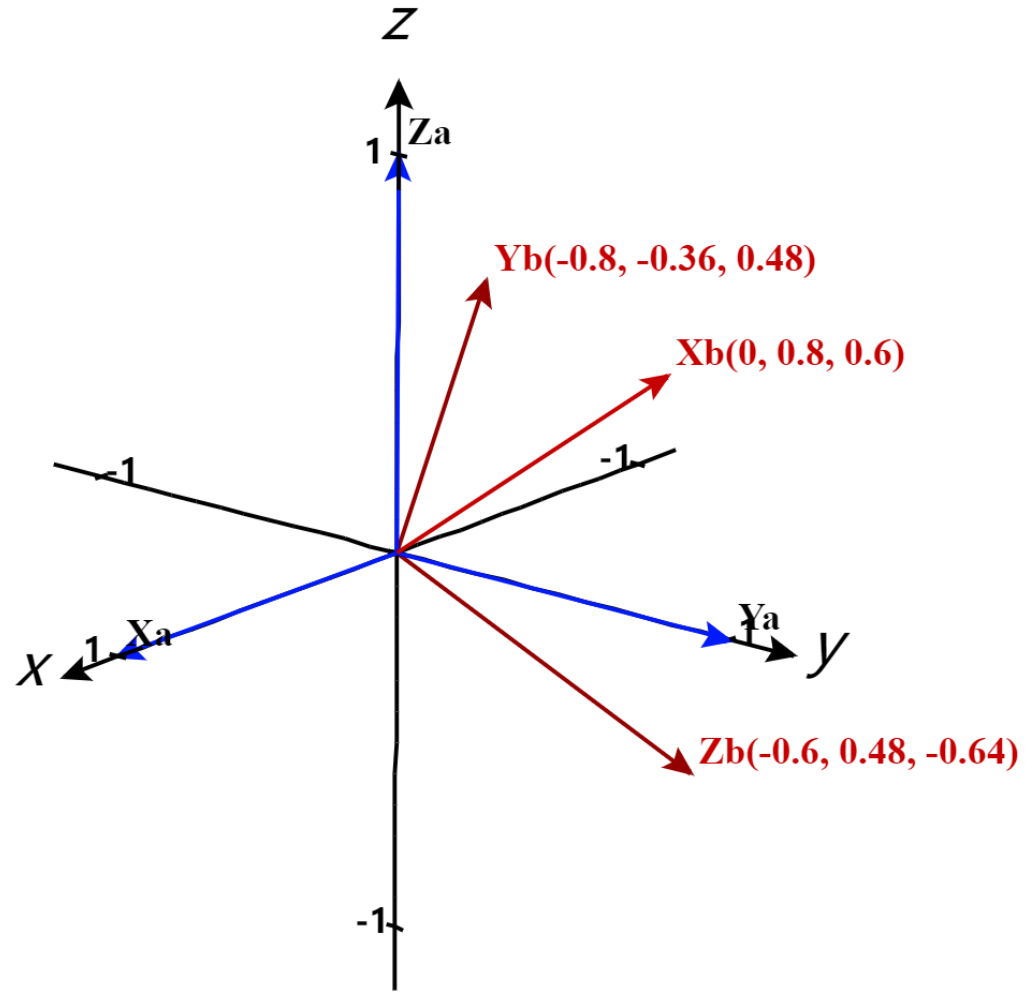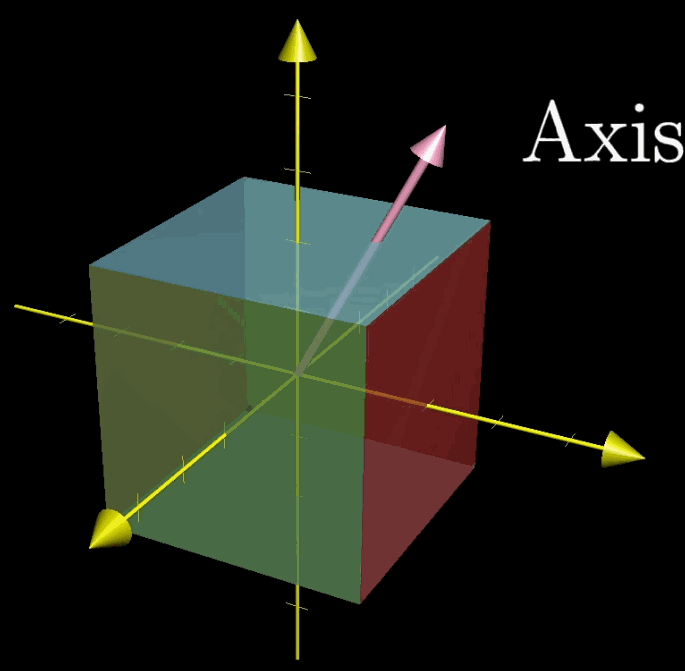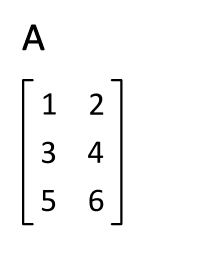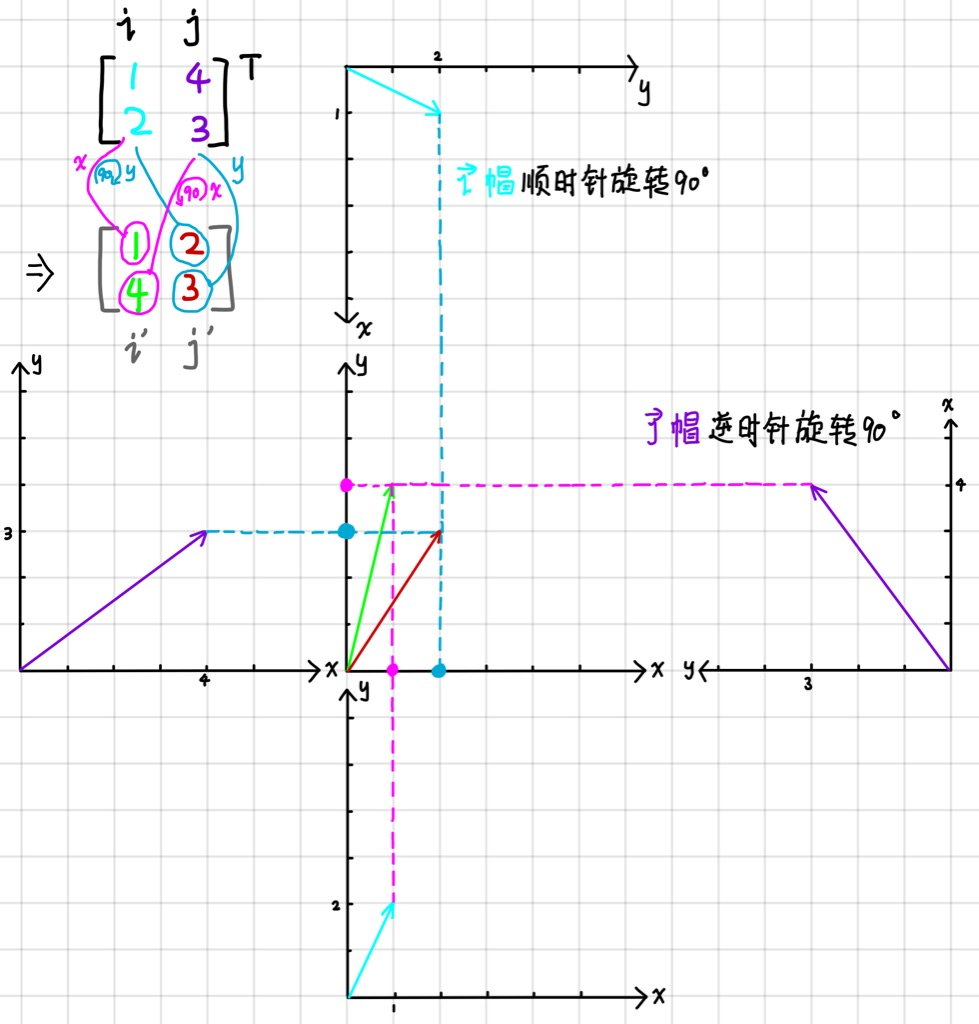【阅读时间】20min 4589 words
【内容简介】从【直观理解】线性代数的本质笔记出发，继续讨论几个线性代数中的概念，正交，正规，正定及转置的直观解释。旨在能帮助读者在看完后不会忘记什么是正交矩阵，什么是正规矩阵，转置部分进行了深入挖掘，希望找出一些几何直观的解释

# 正交矩阵$\mathbf E$ 中的三个基向量分别记为 $\mathbf {X_a}= \left [ \begin{smallmatrix} 1 \\ 0 \\ 0 \end{smallmatrix} \right ]$ ，$\mathbf {Y_a} = \left [ \begin{smallmatrix} 0 \\ 1 \\ 0 \end{smallmatrix} \right ]$，$\mathbf {Z_a} = \left [ \begin{smallmatrix} 0 \\ 0 \\ 1 \end{smallmatrix} \right ]$ ，用下标a来表示。之后对这个矩阵 $\mathbf E$ 应用一个旋转变换，以 $(0,-0.6,0.8)$ 为旋转轴，转90°。得到三个新的向量，用下标b来表示，记为 $\mathbf {X_b}= \left [ \begin{smallmatrix} 0 \\ 0.8 \\ 0.6 \end{smallmatrix} \right ]$ ，$\mathbf {Y_b} = \left [ \begin{smallmatrix} -0.8 \\ -0.36 \\ 0.48 \end{smallmatrix} \right ]$，$\mathbf {Z_b} = \left [ \begin{smallmatrix} -0.6 \\ 0.48 \\ -0.64 \end{smallmatrix} \right ]$

$$\mathbf R^{-1} = \left [ \begin{matrix} 0 & 0.8 & 0.6 \\-0.8 & -0.36 & 0.48 \\-0.6 & 0.48 & -0.64 \end{matrix}\right]$$

# 正定与半正定矩阵

$$\vec v^T \mathbf A \vec v = \vec v^T \lambda \vec v = \lambda \vec v^T \vec v \tag{2-1}$$

$$\vec x^T \vec y > 0 \tag{2-2}$$

$$\cos(\theta) = \frac{\vec x^T \vec y}{\Vert \vec x \Vert * \Vert \vec y \Vert} \hat {\jmath}$$

$\Vert \vec x \Vert \; \Vert \vec y \Vert$ 表示 $\vec x$ 和 $\vec y$的长度，$\theta$ 是它们之间的夹角。根据2-2式，可以得到 $\cos(\theta) > 0$，即它们之间的夹角小于90度

# 矩阵的转置

## 什么是转置## 为什么转置

### 方阵

#### 性质找规律#### 数学计算

$$\mathbf T \mathbf A = \mathbf A^{T} \tag 1$$

$$\mathbf A= \begin{bmatrix} a_{1} & b_{1} \\ a_{2} & b_{2} \\ \end{bmatrix} \quad \mathbf A^T= \begin{bmatrix} a_{1} & a_{2} \\ b_{1} & b_{2} \\ \end{bmatrix} \tag{2}$$

$$\mathbf T = \frac{1}{det(\mathbf A)} \begin{bmatrix} a_{1} b_{2} - a_{2}^2 & a_{1} (a_{2} - b_{1}) \\ b_{2} (b_{1} - a_{2}) & a_{1} b_{2} - b_{1}^2 \\ \end{bmatrix} \tag{3}$$

$$\mathbf T' = \begin{bmatrix} a_{1} b_{2} - a_{2}^2 & a_{1} (a_{2} - b_{1}) \\ b_{2} (b_{1} - a_{2}) & a_{1} b_{2} - b_{1}^2 \\ \end{bmatrix} \tag {4}$$

$$\mathbf T'=\begin{bmatrix} \begin{bmatrix} a_{1} & a_{2} \\ \end{bmatrix} \begin{bmatrix} b_{2} \\ -a_{2} \\ \end{bmatrix} & \begin{bmatrix} a_{1} & a_{2} \\ \end{bmatrix} \begin{bmatrix} -b_{1} \\ a_{1} \\ \end{bmatrix} \\ \begin{bmatrix} b_{1} & b_{2} \\ \end{bmatrix} \begin{bmatrix} b_{2} \\ -a_{2} \\ \end{bmatrix} & \begin{bmatrix} b_{1} & b_{2} \\ \end{bmatrix} \begin{bmatrix} -b_{1} \\ a_{1} \\ \end{bmatrix} \\ \end{bmatrix} \tag{5}$$

$$B'=\begin{bmatrix} {\mathbf a}^{T} \begin{bmatrix} b_{2} \\ -a_{2} \\ \end{bmatrix} & {\mathbf a}^{T} \begin{bmatrix} -b_{1} \\ a_{1} \\ \end{bmatrix} \\ {\mathbf b}^{T} \begin{bmatrix} b_{2} \\ -a_{2} \\ \end{bmatrix} & {\mathbf b}^{T} \begin{bmatrix} -b_{1} \\ a_{1} \\ \end{bmatrix} \\ \end{bmatrix}= \begin{bmatrix} {\mathbf a}\cdot \begin{bmatrix} b_{2} \\ -a_{2} \\ \end{bmatrix} & {\mathbf a}\cdot \begin{bmatrix} -b_{1} \\ a_{1} \\ \end{bmatrix} \\ {\mathbf b}\cdot \begin{bmatrix} b_{2} \\ -a_{2} \\ \end{bmatrix} & {\mathbf b}\cdot \begin{bmatrix} -b_{1} \\ a_{1} \\ \end{bmatrix} \\ \end{bmatrix} \tag{6}$$

• $\mathbf c$ 变换到 $\mathbf A^T_{\hat {\jmath}}$ 为逆时针旋转90°
• $\mathbf d$ 变换到 $\mathbf A^T_{\hat {\imath}}$ 为顺时针旋转90°

$\mathbf c$ 和 $\mathbf d$ 组成的列空间设为 $\mathbf C$，写成公式为

$$\mathbf C = \begin{bmatrix}\mathbf c & \mathbf d\end{bmatrix} = \begin{bmatrix} b_2 & -b_1 \\ -a_2 & a_1\end{bmatrix} \tag{7}$$

$$\mathbf A\mathbf C= \begin{bmatrix} a_{1} & b_{1} \\ a_{2} & b_{2} \\ \end{bmatrix} \cdot \begin{bmatrix} b_{2} & -b_{1} \\ -a_{2} & a_{1} \\ \end{bmatrix} = \begin{bmatrix} det(\mathbf A) & 0 \\ 0 & det(\mathbf A) \\ \end{bmatrix} =det(\mathbf A) \mathbf I \tag{8}$$

$$\mathbf C = \mathbf A^{-1} det(\mathbf A) \tag{9}$$

$$\mathbf T'=\begin{bmatrix} \begin{bmatrix} \begin{bmatrix} a_{1} & b_{1} \\ a_{2} & b_{2} \\ \end{bmatrix} & \begin{bmatrix} b_{2} \\ -a_{2} \\ \end{bmatrix} \end{bmatrix} \\ \begin{bmatrix} \begin{bmatrix} a_{1} & b_{1} \\ a_{2} & b_{2} \\ \end{bmatrix} & \begin{bmatrix} -b_{1} \\ a_{1} \\ \end{bmatrix} \end{bmatrix} \end{bmatrix} = \begin{bmatrix} \begin{bmatrix} \begin{bmatrix} a_{1} & b_{1} \\ a_{2} & b_{2} \\ \end{bmatrix} & \mathbf c \end{bmatrix} \\ \begin{bmatrix} \begin{bmatrix} a_{1} & b_{1} \\ a_{2} & b_{2} \\ \end{bmatrix} & \mathbf d \end{bmatrix} \end{bmatrix} \tag{10}$$

\begin{align} \mathbf T' & = \begin{bmatrix} \begin{bmatrix} a_{1} & a_{2} \\ \end{bmatrix} & \begin{bmatrix} b_{2} & -b_{1} \\ -a_{2} & a_{1} \\ \end{bmatrix} \\ \begin{bmatrix} a_{2} & b_{2} \\ \end{bmatrix} & \begin{bmatrix} b_{2} & -b_{1} \\ -a_{2} & a_{1} \\ \end{bmatrix} \\ \end{bmatrix} = \begin{bmatrix} \mathbf a^{T} & \begin{bmatrix} \mathbf c & \mathbf d \\ \end{bmatrix} \\ \mathbf b^{T} & \begin{bmatrix} \mathbf c & \mathbf d \\ \end{bmatrix} \\ \end{bmatrix} \\ & = \begin{bmatrix} \mathbf c^{T}\mathbf C \\ \mathbf d^{T}\mathbf C \\ \end{bmatrix} = det(\mathbf A) \begin{bmatrix} \mathbf a^{T}\mathbf A^{-1} \\ \mathbf b^{T}\mathbf A^{-1} \\ \end{bmatrix} \end{align} \tag{11}

$$\mathbf T= \begin{bmatrix} \mathbf a^T \mathbf A^{-1} \\ \mathbf b^T \mathbf A^{-1} \end{bmatrix} \tag{12}$$

$$AA^T = \begin{bmatrix} a_1 & b_1 \\ a_2 & b_2\end{bmatrix}\begin{bmatrix} a_1 & a_2 \\ b_1 & b_2\end{bmatrix} = \begin{bmatrix} a_1^2 + b_1^2 & a_1a_2+b_1b_2 \\ a_1a_2+b_1b_2 & a_2^2 + b_2^2\end{bmatrix} \tag{13}$$

$$AA^{T} = R_{AA^{T}} \Lambda_{AA^{T}} (R^{-1})_{AA^{T}} \tag{14}$$

$$A^{T} = A^{-1} R_{AA^{T}} \Lambda_{AA^{T}} (R^{-1})_{AA^{T}} \tag{15}$$

$$R_{AA^{T}}^{'} = \begin{bmatrix} cos \alpha & -sin \alpha \\ sin \alpha & cos \alpha \\ \end{bmatrix} \tag{16}$$

$$A^{T}=A^{-1} R_{AA^{T}}^{'} \begin{bmatrix} -1 & 0 \\ 0 & 1 \\ \end{bmatrix} \Lambda_{AA^{T}} R_{AA^{T}}^{'} \begin{bmatrix} -1 & 0 \\ 0 & 1 \\ \end{bmatrix} \tag{17}$$

$$A^{T}=A^{-1} R_{\alpha} M_y \Lambda R_{\alpha} M_y \tag{18}$$

$M_y$ 表示的是关于y轴对称
$R_\alpha$ 表示逆时针旋转 $\alpha$ 度
$\Lambda$ 表示一种伸缩变换

# 总结

• $\mathbf A \mathbf A^T$ 的对称特性非常有用
• 正交 = 旋转 = 垂直，并且逆等于转置
• 正规矩阵的定义和转置息息相关，但是从形式上来看，约束条件是逐步变弱的，其实这些特征都是描述了空间一个变换的一些变化模式，比如旋转，伸缩的特殊模式，只需要有一个基本的直观理解，在机器学习领域就已经足够用了